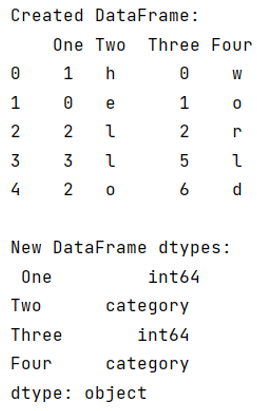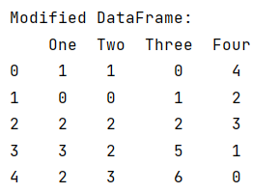# Convert categorical data in pandas dataframe

Given a Pandas DataFrame, we have to convert categorical data in it.
Submitted by Pranit Sharma, on June 28, 2022

Pandas is a special tool that allows us to perform complex manipulations of data effectively and efficiently. Inside pandas, we mainly deal with a dataset in the form of DataFrame. DataFrames are 2-dimensional data structures in pandas. DataFrames consist of rows, columns, and the data. The Data inside the DataFrame can be of any type.

Here, we are going to learn how to convert categorical data in pandas DataFrame? Categorical data is a type of data that has some certain category or characteristic, the value of categorical data is not a single value, rather it consists of classified values, for example, an email can be considered as spam or not spam, if we consider 1 as spam and 0 as not spam, we have a classified data in the form of 0 or 1, this is called categorical data. We will pass a string called 'category' inside the astype() method to first make the data categorical.

To work with pandas, we need to import pandas package first, below is the syntax:

```import pandas as pd
```

Let us understand with the help of an example,

## Python code to convert categorical data in pandas dataframe

```# Importing pandas package
import pandas as pd

# Creating a dictionary
d = {
'One':[1,0,2,3,2],
'Two':list('hello'),
'Three':[0,1,2,5,6],
'Four':list('world')
}

# Creating dataframe
df = pd.DataFrame(d)

# Display DataFrame
print("Created DataFrame:\n",df,"\n")

# Changing dtypes of column Two and Four
df['Two'] = df['Two'].astype('category')
df['Four'] = df['Four'].astype('category')

# Display dtypes of df
print("New DataFrame dtypes:\n",df.dtypes,"\n")
```

Output:Now, we will select all those columns whose data type is categorical and then use cat.codes() method.

```# Changing dtypes of column Two and Four
df['Two'] = df['Two'].astype('category')
df['Four'] = df['Four'].astype('category')

# Display dtypes of df
print("New DataFrame dtypes:\n",df.dtypes,"\n")

# Selecting columns having dtpe category
category = df.select_dtypes(['category']).columns

# Converting category data into df
df[category] = df[category].apply(lambda x: x.cat.codes)

# Display modified DataFrame
print("Modified DataFrame:\n",df)
```

Output:Top MCQs